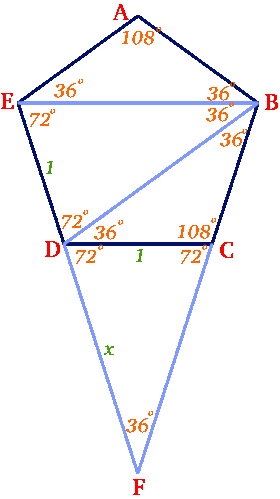#### You may also like### At a Glance

The area of a regular pentagon looks about twice as a big as the pentangle star drawn within it. Is it?### Golden Triangle

Three triangles ABC, CBD and ABD (where D is a point on AC) are all isosceles. Find all the angles. Prove that the ratio of AB to BC is equal to the golden ratio.### Darts and Kites

Explore the geometry of these dart and kite shapes!

# Pentakite

##### Age 14 to 18Challenge LevelOliver from Madras College sent a good solution to this question. From the fact that the angles of a regular pentagon are $108^{\circ}$ all the angles marked in the diagram can be found and we leave the reader to prove these results. Triangles $CDF$ and $EDB$ are congruent because they have the same angles and $CD$ and $ED$ are equal in length. If the sides of the regular pentagon are 1 unit and $FD = x$ units then, because $CDF$ and $EDB$ are congruent isosceles triangles it follows that $BE = x$ units. Triangles $BEF$ and $EDB$ are similar, hence $$\frac{x}{1} = \frac{x + 1}{x}$$ Simplifying this expression gives the equation: $x^2 - x - 1 = 0$. Solving this equation (and taking the positive root as $x$ is positive) $x = (1 + \sqrt{5})/2$ which is equal to the golden ratio. Interestingly this gives us an exact value for $\cos 36^{\circ}$ because $2 \cos 36^{\circ} = x$, so $\cos 36^{\circ} = (1 + \sqrt{5})/4$.